# Solution assignment 07 Equations with fractions

### Assignment 7

For which value(s) of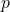does the following equation have two different solutions?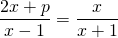### Solution

Cross-multiplication yields: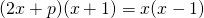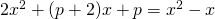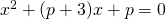This is a quadratic equation in. In order to find out whether this equation has two different solutions we look at the discriminant which has to be greater than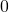: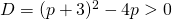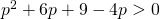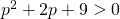The graph of the function inin the left-hand side is an 'opens up' parabola lying above the horizontal axis because the discriminant is less than: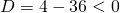We may conclude that the inequality inholds for alland thus that the equation inhas two solutions for all values of.

Note that we used a discriminant twice. In one case we needed the discriminant to determine whether the equation inhas two different solutions. In the other case we needed the discriminant to determine whether the graph of the function inlies above the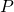-axis.